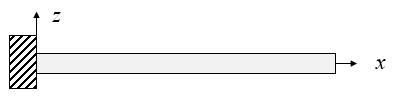﻿ Cantilever beam513.160 Microelectronics and Micromechanics

## Cantilever beamFor a cantilever beam that is fixed at $x=0$ and free at $x=L_x$, the boundary conditions are,

$\begin{equation} w = 0\hspace{0.5cm}\text{and}\hspace{0.5cm}\frac{\partial w}{\partial x}=0\hspace{0.5cm}\text{at}\hspace{0.5cm}x=0, \end{equation}$ $\begin{equation} \frac{\partial^2 w}{\partial x^2} = 0\hspace{0.5cm}\text{and}\hspace{0.5cm}\frac{\partial^3 w}{\partial x^3}=0\hspace{0.5cm}\text{at}\hspace{0.5cm}x=L_x. \end{equation}$

In this case, the normal mode solutions to the Euler-Lagrange equation for an unloaded cantilever beam are,

$\begin{equation} w_n(x,t) = A_n\left(\cosh (k_nx)-\cos (k_nx)+\frac{\left(\cos (k_nL_x)+\cosh (k_nL_x)\right)\left(\sin (k_nx)-\sinh (k_nx)\right)}{\sin (k_nL_x)+\sinh (k_nL_x)}\right)\hspace{1cm}n=1,2,3,\cdots \end{equation}$

where the allowed wavenumbers are determined numerically by the condition, $\cosh(k_nL_x)\cos(k_nL_x) +1 =0$. The first few solutions are $k_1L_x=1.875,\, k_2L_x = 4.694,\, k_3L_x = 7.855,\, k_4L_x = 10.9955,\, k_5L_x = 14.137,\,\cdots$

The corresponding frequencies are given by the formula,

$\begin{equation} \omega = \sqrt{\frac{EI}{\mu}}k^2. \end{equation}$

The figure below shows the form of the first four cantilever modes. These are small amplitude oscillations. Notice that the vertical scale is expanded compared to the horizontal scale.

 $w$ $x/L_x$

Animation speed:

If a static point force is applied to the end of the cantilever, $q=F\delta (x=L_x)$, then the displacement along the beam is,

$\begin{equation} w(x) = \frac{F}{6EI}(3L_xx^2-x^3), \end{equation}$

and the effective spring constant for a point force applied to the end of the cantilever is $K_{eff}=3EI/L_x^3$. The restoring force that opposes the applied force is $-K_{eff}w$.

If the load $q$ is constant along a cantilever beam, the displacement is,

$\begin{equation} w(x) = \frac{qx^2(6L_x^2-4L_xx+x^2)}{24EI}, \end{equation}$

and the displacement at the end of the beam is $w(x=L_x)=\frac{qL^4}{8EI}$.